NEET  >  Test: Common Forces in Mechanics

# Test: Common Forces in Mechanics

Test Description

## 20 Questions MCQ Test Physics Class 11 | Test: Common Forces in Mechanics

Test: Common Forces in Mechanics for NEET 2023 is part of Physics Class 11 preparation. The Test: Common Forces in Mechanics questions and answers have been prepared according to the NEET exam syllabus.The Test: Common Forces in Mechanics MCQs are made for NEET 2023 Exam. Find important definitions, questions, notes, meanings, examples, exercises, MCQs and online tests for Test: Common Forces in Mechanics below.
Solutions of Test: Common Forces in Mechanics questions in English are available as part of our Physics Class 11 for NEET & Test: Common Forces in Mechanics solutions in Hindi for Physics Class 11 course. Download more important topics, notes, lectures and mock test series for NEET Exam by signing up for free. Attempt Test: Common Forces in Mechanics | 20 questions in 20 minutes | Mock test for NEET preparation | Free important questions MCQ to study Physics Class 11 for NEET Exam | Download free PDF with solutions
 1 Crore+ students have signed up on EduRev. Have you?
Test: Common Forces in Mechanics - Question 1

### A train is moving along a horizontal track. A pendulum suspended from the roof of the train makes an angle of 5° with the vertical. The acceleration of the train is

Detailed Solution for Test: Common Forces in Mechanics - Question 1

Making the free body diagram of the pendulum bob we get
T.cosθ = mg
T.sinθ = ma (applying the concept of pseudo force)
By dividing the above two equations we get
tanθ = a/g
Thus we get a = g.tanθ
= 10 x tan 5°
= 0.87 ms-2

Test: Common Forces in Mechanics - Question 2

### A body of weight 100 N is suspended with the help of strings as shown in figure.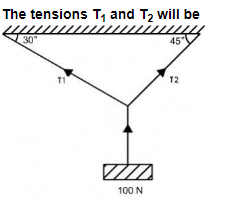Detailed Solution for Test: Common Forces in Mechanics - Question 2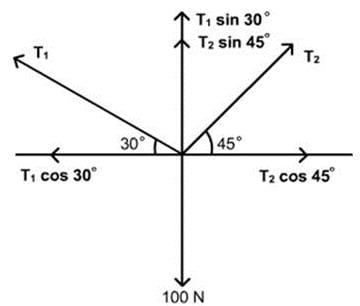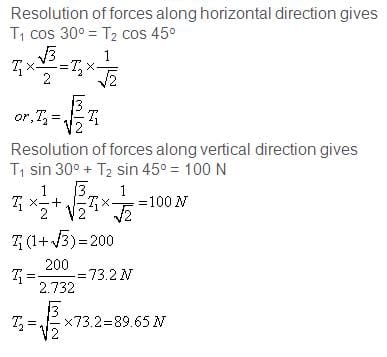Test: Common Forces in Mechanics - Question 3

### For a body along the inclined plane, the normal reaction acts

Detailed Solution for Test: Common Forces in Mechanics - Question 3

Normal force is the perpendicular component of the reaction force wrt the surface and thus it always acts perpendicularly to the surface.

Test: Common Forces in Mechanics - Question 4

Two forces F1 and F2 are acting on a particle. The particle will remain at rest if two forces are

Detailed Solution for Test: Common Forces in Mechanics - Question 4

For an object at rest to be at rest, no net acceleration must act upon it, which implies no net force. Thus the two forces need to nullify each other, which is only possible if both are equal but opposite.

Test: Common Forces in Mechanics - Question 5

If no resultant force acts on a body then the body will be in

Detailed Solution for Test: Common Forces in Mechanics - Question 5

What do you think about Newton's 1st law, he was said that " every body have nature to maintain inertia of rest or motion untill there is not net force applied on that body". means body will be earlier state (no change in state) when net force applied on that body equals zero.
I know, you thought answer is option (a). but this is not true. for better understanding, Let's take. an example. a body moves with uniform velocity then, net force applied on body = 0 because acceleration of body is zero . but here you see body is not in rest . it is in motion. it is in earlier state . its state doesn't change.

Test: Common Forces in Mechanics - Question 6

A body is at rest though forces act on it. Then

Detailed Solution for Test: Common Forces in Mechanics - Question 6

“Every body continues to be in its state of rest or of uniform motion in a straight line unless compelled by an unbalanced external force.” It is simply expressed as if the net external force on a body is zero, its acceleration is zero. Acceleration can be non-zero only if there is a net external force on the body.

Test: Common Forces in Mechanics - Question 7

In mechanics, if the net external force on the particle is zero, then the particle is said to be in state of

Detailed Solution for Test: Common Forces in Mechanics - Question 7

In mechanics, if the net external force on the particle is zero, then the particle is said to be in state of equilibrium. By definition.

Test: Common Forces in Mechanics - Question 8

A book is lying on the table. What is the angle between the action of the book on the table and reaction of table on the book?

Detailed Solution for Test: Common Forces in Mechanics - Question 8

Action Force and Reaction Force are in opposite directions.
So, the angle between them is 180º

Test: Common Forces in Mechanics - Question 9

A mass of 6 kg is suspended by a rope of length 2 m from a ceiling. A force of 50 N in the horizontal direction is applied at the mid-point of the rope, as shown. What is the angle the rope makes with the vertical in equilibrium?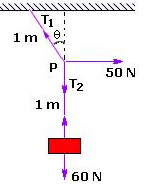Detailed Solution for Test: Common Forces in Mechanics - Question 9

Making the free body diagram of the body we get
T1.cosθ = T2 = mg = 60
T1.sinθ = 50N
By dividing the above two equations we get
tanθ = 5/6
Thus we get θ = 40°

Test: Common Forces in Mechanics - Question 10

Which of the conditions shows that the particles are in equilibrium state for two forces F1 and F2 acting on the body?

Detailed Solution for Test: Common Forces in Mechanics - Question 10

At equilibrium condition net force on body is 0
F1 + F2 = 0
F1 = -F2

Test: Common Forces in Mechanics - Question 11

Which of the following forces is not considered as a contact force in Mechanics?

Detailed Solution for Test: Common Forces in Mechanics - Question 11

The force exerted by the earth on a body is called gravitational force. Actually this force exists between any two bodies in the universe.This force is always of attraction. e.g. When a body is dropped from a height it moves in downward direction towards the Earth with increasing speed (with constant acceleration). This constant acceleration by which all bodies fall down is called acceleration due to gravity. Its value is 9.8 m/s' (approx 10 m/s' )on the surface of the earth. e.g. i) A fruit from tree falls down;ii) Water falls down on a ground from a tap.iii) We feel the weight of bucket full of water holding in our hand.

Test: Common Forces in Mechanics - Question 12

Weight and normal reaction forces

Detailed Solution for Test: Common Forces in Mechanics - Question 12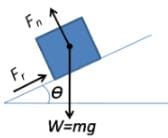In a simple case such as an object resting upon a table, the normal force on the object is equal but in opposite direction to the gravitational force applied on the object (or the weight of the object), that is, , where m is mass, and g is the gravitational field strength (about 9.81 m/s2 on Earth).

Test: Common Forces in Mechanics - Question 13

The contact forces of mechanics are fundamentally derived from

Detailed Solution for Test: Common Forces in Mechanics - Question 13

Friction is a surface phenomenon. When two surfaces have irregularities that come in contact do they get interlocked  preventing them against sliding each other. So, frictional force is a contact force dependent on the surface.
Gravitational force, electrostatic force and magnetic force does not depend on the surface.

Test: Common Forces in Mechanics - Question 14

The minimum angle that an inclined plane makes with the horizontal when a body placed on it just begins to slide down is called

Detailed Solution for Test: Common Forces in Mechanics - Question 14

The minimum angle that an inclined plane makes with the horizontal when a body placed on it just begins to slide down is called angle of repose.

Test: Common Forces in Mechanics - Question 15

A body in equilibrium

Detailed Solution for Test: Common Forces in Mechanics - Question 15

A body is said to be in equilibrium only when the net resultant of all external forces applied on the body becomes zero. It does not depend upon any of the internal forces because for any system internal forces exist in pairs because of Newton's third law  and hence they result in null.

Test: Common Forces in Mechanics - Question 16

Spring force is a restoring force.

Detailed Solution for Test: Common Forces in Mechanics - Question 16

The force exerted by a spring is called a restoring force; it always acts to restore the spring toward equilibrium. In Hooke's law, the negative sign on the spring's force means that the force exerted by the spring opposes the spring's displacement.

Test: Common Forces in Mechanics - Question 17

The forces F1, F2, and F3 are acting on a particle of mass m, such that F2 and F3are mutually perpendicular and under the effect of F1, F2, and F3 , the particle remains stationary. What will be the acceleration of the particle, if the force F1 is removed?

Detailed Solution for Test: Common Forces in Mechanics - Question 17

Concept: Forces.The particle is a stationary under the effect of forces F1, F2 and F3.
This shows that force F1 is equal and opposite to the resultant of forces F2 and F3.
Hence, if the force F1 is removed the particle will move under the action of the force -ve F1 and the acceleration will the particle will be,a =-F1/m.

Test: Common Forces in Mechanics - Question 18

Tension on an almost massless rope being pulled by two boys, one small and one big, will be

Detailed Solution for Test: Common Forces in Mechanics - Question 18

Because the Tension for rope at each point is always the same.

Test: Common Forces in Mechanics - Question 19

Which of the following cannot be regarded as yet another kind of force?

Detailed Solution for Test: Common Forces in Mechanics - Question 19

if an object is moving in a horizontal circle at constant speed, the centripetal force does not do any work and cannot alter the total mechanical energy of the object. For the reason, the kinetic energy and therefore the speed of the object will remain constant.

Test: Common Forces in Mechanics - Question 20

The component of contact force normal to the surfaces in contact is called

Detailed Solution for Test: Common Forces in Mechanics - Question 20

The component of contact force normal to the surfaces in contact is called normal reaction. The component parallel to the surfaces in contact is called friction.

## Physics Class 11

127 videos|464 docs|210 tests
 Use Code STAYHOME200 and get INR 200 additional OFF Use Coupon Code
Information about Test: Common Forces in Mechanics Page
In this test you can find the Exam questions for Test: Common Forces in Mechanics solved & explained in the simplest way possible. Besides giving Questions and answers for Test: Common Forces in Mechanics, EduRev gives you an ample number of Online tests for practice

## Physics Class 11

127 videos|464 docs|210 tests

### How to Prepare for NEET

Read our guide to prepare for NEET which is created by Toppers & the best Teachers